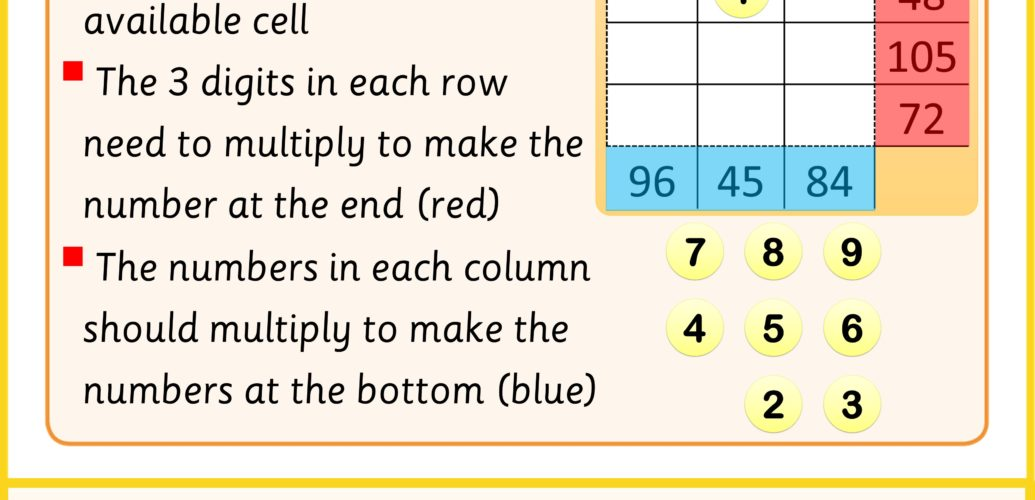# Turning times tables inside out

### Challenge On Boarding – Is it a factor?

#### Divisibility

Take a number, say 45.  Just looking at it can get you some information about its factors.  For example, it is an odd number, which just means it does not have 2 as a factor.

We can explore divisibility patterns using a 100 square.

Start with the 2 times table.  You can do this by counting in 2s and colouring in only those numbers e.g. 2, 4, 6, 8 etc.

Then try other numbers.

Which numbers do you think will show the clearest patterns?

What do you notice about multiples of 4?

How would you describe the pattern that multiples of 9 make?

### Challenge On Boarding – Divisibility Rules

#### Rules by the numbers

There are some factors that make it easy to tell if a number is a multiple of that factor or not.

2, for example, you can tell if a number is a multiple of 2 because the ones digit will be a multiple of 2.  So if a number ends in any of the following:  0, 2, 4, 6, 8 then it’s a multiple of 2 or we can say even.

5 as a factor also makes it very easy.  You can tell if a number is a multiple of 5 or not by looking at the last digit.

3 and 9 have quite a different pattern.  Have you noticed the visual pattern on the hundred square?  Well there is a way to tell just from the possible multiple.

This method is based on finding digital roots.

#### Digital Roots

To find a numbers digital roots, you have to separate the digits of the number and add them together.

Either,

the total is a multiple of 3 (or 9) – Your number is a multiple of 3 (or 9)

Or it isn’t – Your number isn’t a multiple of 3 or 9

Or you can’t tell yet – take the digital roots of that number and check again

#### Combining techniques

6 is both a multiple of 2 and 3, so you can use both the multiple of 3 test and the multiple of 2 test to see if a number is a multiple of 6

### Challenge On Boarding – Using odd and even

#### Is 2 a factor or not?

Really to do this puzzle, you need to be able to see if a given number is a factor or not.  For example, you have the number 252.  You want to know which of the numbers from 1 to 9 are factors.

It’s easier to start off testing whether the number is a factor of two or not.

We can do this by making our own grid and just decide if the number is odd or even

Even = the number’s a multiple of 2

Odd = the number’s not a multiple of 2

What happens when you multiply an odd number by an even number?  What examples could help you decide?

Try to find examples of the following by writing in example numbers and see you get the same odd and even patterns every time.

This suggests that Even times Odd is always Even.  We haven’t proved this but for now, a few examples will do.  How many different combinations of Odd and Even can you find?  Here are 3 to start you off.

### Now, Try the Challenges

Challenge 1 – Odd and even patterns

Challenge 2 – How many different products?

Challenge 3 – Complete the inside out multiplication grid

Download or share all of these challenges with this pdf and let us know how you got on with it by tweeting using #momentofmaths and start to see Every moment is a #momentofmaths.